您可以捐助，支持我们的公益事业。 1元 10元 50元 认证码：必填求知 文章 文库 Lib 视频 iPerson 课程 认证 咨询 工具 讲座 Modeler Code要资料订阅捐助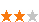3024 次浏览     评价： 好 中 差
2018-11-20

 编辑推荐: 本文来自于csdn，本文章主要是对真实数据进行实战，手把手带你走一遍使用机器学习对真实数据进行处理的全过程。

Scikit-learn集成了很多机器学习需要使用的函数，学习Scikit-learn能简洁、快速写出机器学习程序。并且通过代码更加深入的了解机器学习模型，学习如何处理数据，如何选择模型，如何选择和调整模型参数。

1、推荐安装Anaconda（集成Python和很多有用的Package）

2、编辑器：Spyder 或 Pycharm 或 Jupyter Notebook

1、下载数据

 import os import tarfile from six.moves import urllib DOWNLOAD_ROOT = "https://raw.githubusercontent.com/ageron/handson-ml/master/" HOUSING_PATH = "datasets/housing" HOUSING_URL = DOWNLOAD_ROOT + HOUSING_PATH + "/housing.tgz" def fetch_housing_data(housing_url=HOUSING_URL, housing_path=HOUSING_PATH): if not os.path.isdir(housing_path): os.makedirs(housing_path) tgz_path = os.path.join(housing_path, "housing.tgz") urllib.request.urlretrieve(housing_url, tgz_path) housing_tgz = tarfile.open(tgz_path) housing_tgz.extractall(path=housing_path) housing_tgz.close() fetch_housing_data()

2、读入数据

3、观察数据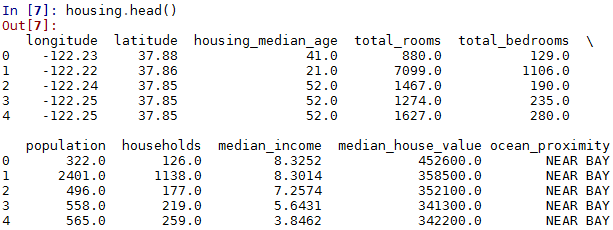info()输出每个特征的元素总个数以及类型信息等

info()可以查看每个特征的元素总个数，因此可以查看某个特征是否存在缺失值。还可以查看数据的类型以及内存占用情况。

 housing.info()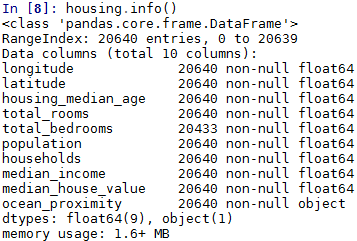value_counts()统计特征中每个元素的总个数

value_counts()一般用在统计有有限个元素的特征（如标签label，地区等）

 housing["ocean_proximity"].value_counts()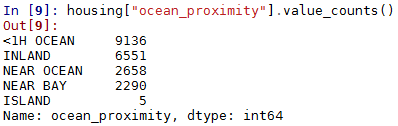describe()可以看实数特征的统计信息

describe()可以看实数特征的最大值、最小值、平均值、方差、总个数、25%，50%，75%小值。

 housing.describe()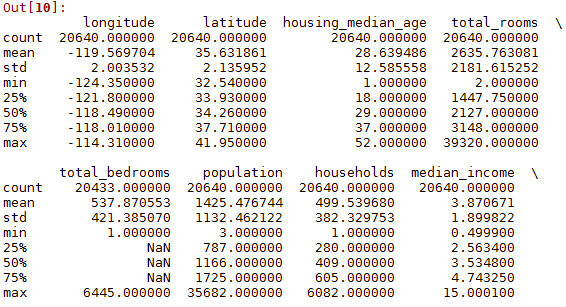hist()输出实数域的直方图

 import matplotlib.pyplot as plt housing.hist(bins=50, figsize=(20,15)) plt.show()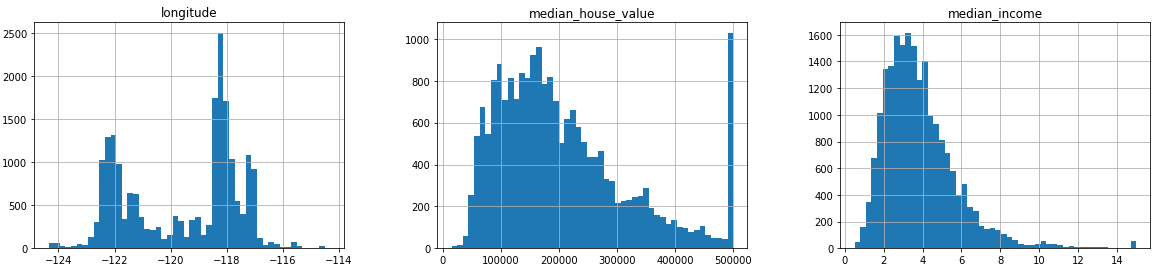4、分开训练和测试集

 import numpy as np def split_train_test(data, test_ratio): shuffled_indices = np.random.permutation(len(data)) test_set_size = int(len(data) * test_ratio) test_indices = shuffled_indices[:test_set_size] train_indices = shuffled_indices[test_set_size:] return data.iloc[train_indices], data.iloc[test_indices] train_set, test_set = split_train_test(housing, 0.2) print(len(train_set), "train +", len(test_set), "test")

 def test_set_check(identifier, test_ratio, hash): return hash(np.int64(identifier)).digest()[-1] < 256 * test_ratio def split_train_test_by_id(data, test_ratio, id_column, hash=hashlib.md5): ids = data[id_column] in_test_set = ids.apply(lambda id_: test_set_check(id_, test_ratio, hash)) return data.loc[~in_test_set], data.loc[in_test_set] housing_with_id = housing.reset_index() # adds an `index` column train_set, test_set = split_train_test_by_id(housing_with_id, 0.2, "index")

 housing_with_id["id"] = housing["longitude"] * 1000 + housing["latitude"] train_set, test_set = split_train_test_by_id(housing_with_id, 0.2, "id")

 from sklearn.model_selection import train_test_split train_set, test_set = train_test_split(housing, test_size=0.2, random_state=42)

 housing["income_cat"] = np.ceil(housing["median_income"] / 1.5) housing["income_cat"].where(housing["income_cat"] < 5, 5.0, inplace=True)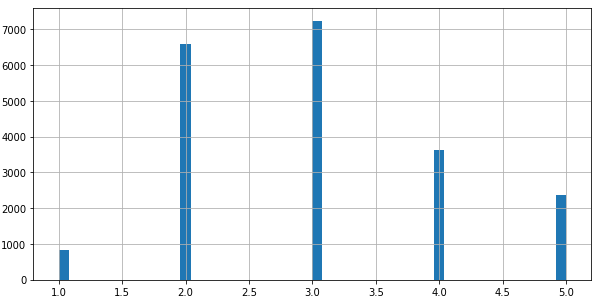from sklearn.model_selection import StratifiedShuffleSplit split = StratifiedShuffleSplit(n_splits=1, test_size=0.2, random_state=42) for train_index, test_index in split.split(housing, housing["income_cat"]): strat_train_set = housing.loc[train_index] strat_test_set = housing.loc[test_index]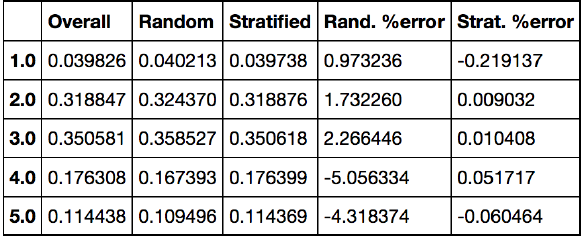for set in (strat_train_set, strat_test_set): set.drop(["income_cat"], axis=1, inplace=True)3024 次浏览     评价： 好 中 差订阅捐助
 相关文章 我们该如何设计数据库 数据库设计经验谈 数据库设计过程 数据库编程总结
 相关文档 数据库性能调优技巧 数据库性能调整 数据库性能优化讲座 数据库系统性能调优系列